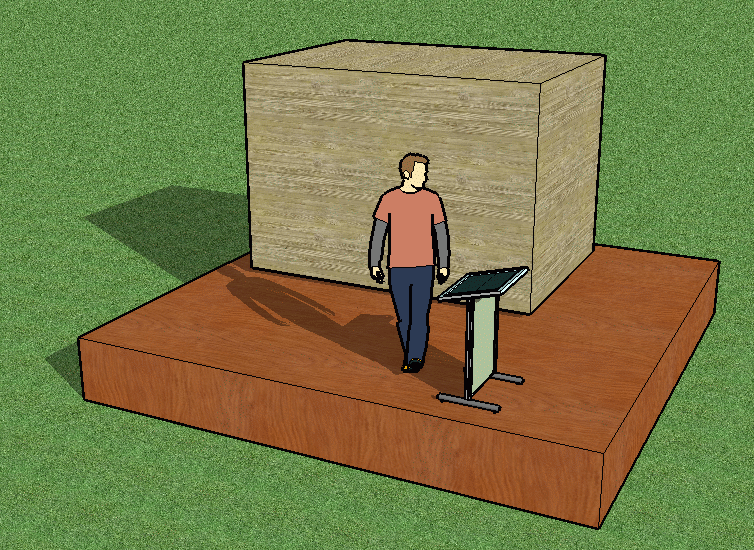SEARCH HOMEMath Central Quandaries & QueriesQuestion from Kimberly, a parent: How do you convert 487 cf into box?Hi Kimberly. Many ways.

Any three numbers that you can multiply together to get 487 can be the dimensions in feet of a rectangular box. You could make a large square box that is really thin, like a pizza box, hold that much space, or you could make a basic cube (all sides equal to about 7.9 feet).The two wooden boxes shown each have about 487 cubic feet of volume.

If this is confusing, think about area. If you want a 200 square foot garden, you could make one that is 20 feet by 10 feet or make it 40 feet by 5 feet or even 8 feet by 25 feet if you like. All would have 200 square feet in area. The same principle applies to three-dimensional shapes likes boxes and their volumes.

The easiest way I can think of for making your box is to first choose two numbers that you find convenient for the dimensions of one side of your box (perhaps the bottom), then divide these into the space you need to find the third dimension.

For example, I can choose a bottom that is 7 feet wide and 10 feet long. If I want the box to hold 487 cubic feet, then I divide: 487 ÷ 7 ÷ 10 = 6.96 feet. So I need to make the height 6 ft, 11½ in.

Hope this helps,
Stephen La Rocque.Math Central is supported by the University of Regina and The Pacific Institute for the Mathematical Sciences.